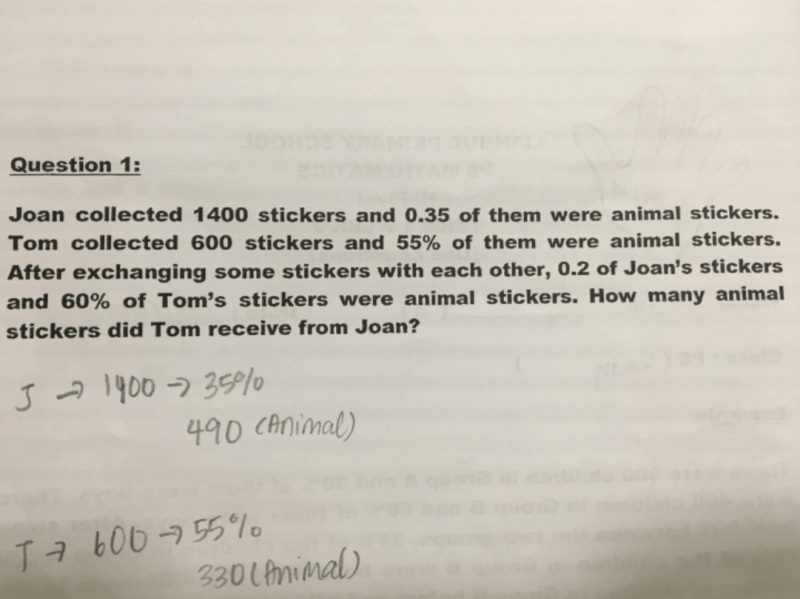# QuestionDear friends,

How to solve above question?

Thanks!!

1 Answer

# Answer

Key concepts:

• Treat each percentage point as a single unit/part
• Identify the constant total (of animal stickers)

Total stickers → 1400 + 600 = 2000
Joan’s animal stickers at first → 35% of 1400 = 490
Tom’s animal stickers at first → 55% of 600 = 330
Total animal stickers → 490 + 330 = 820

100% of John’s stickers + 100% of Tom’s stickers = 2000
20% of John’s stickers (final) + 60% of Tom’s stickers (final) = 820

 Joan Tom Total Initial and final (Total) 100% 100% 2000 Final (Animal only) 20% 60% 820

Multiply the second row by 5 to get equal units:

 100% 300% 4100

Comparing the first and third column:

200% of Tom’s stickers in the end = 4100 – 2000 = 2100
100% of Tom’s stickers in the end = 2100 ÷ 2 = 1050
60% of Tom’s stickers in the end = 60/100 × 1050 = 630 (animal stickers only)

Since Tom only had 330 animal stickers at the start, this means that after the exchange, he had a net increase of (630 – 330 = 300) animal stickers.

1 Reply 1 Like ✔Accepted Answer

Excellent. Thanks a lot for your response. Very helpful!!!

0 Replies 0 Likes